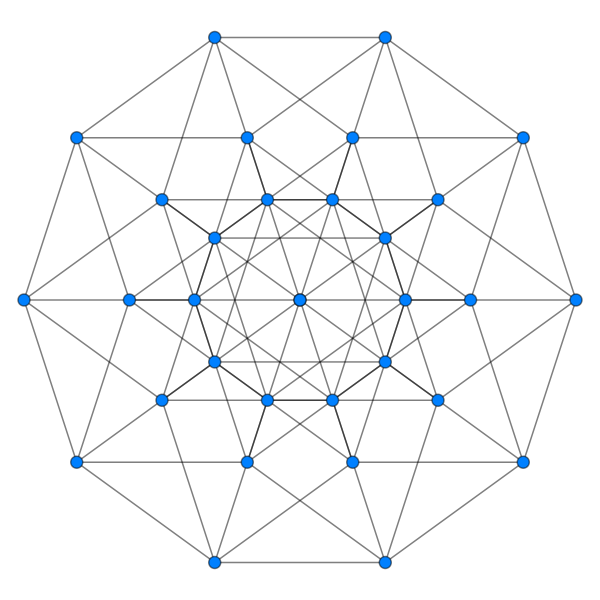# 5-D on 2-D

Geometry Level 5A hypercube in 5 dimensions has $32 = 2 ^ 5$ vertices of the form $( \pm 1, \pm 1, \pm 1, \pm 1, \pm 1)$.

For each pair of distinct vertices, we connect them up with a line segment and mark the midpoint.

How many (distinct) midpoints have been marked?

×